# Geometry Lesson 7 4 Parallel Lines and Proportional

• Slides: 17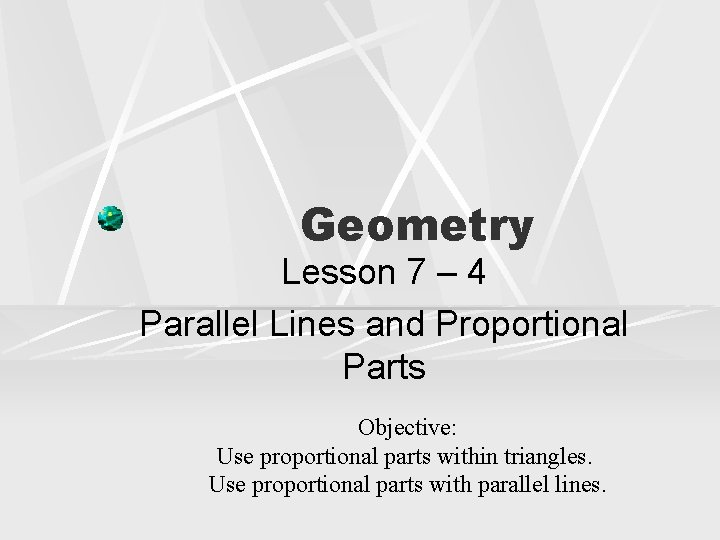Geometry Lesson 7 – 4 Parallel Lines and Proportional Parts Objective: Use proportional parts within triangles. Use proportional parts with parallel lines.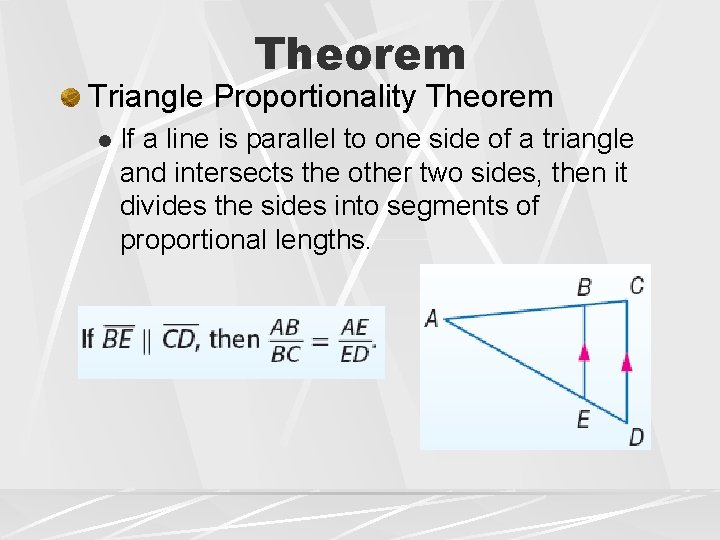Theorem Triangle Proportionality Theorem l If a line is parallel to one side of a triangle and intersects the other two sides, then it divides the sides into segments of proportional lengths.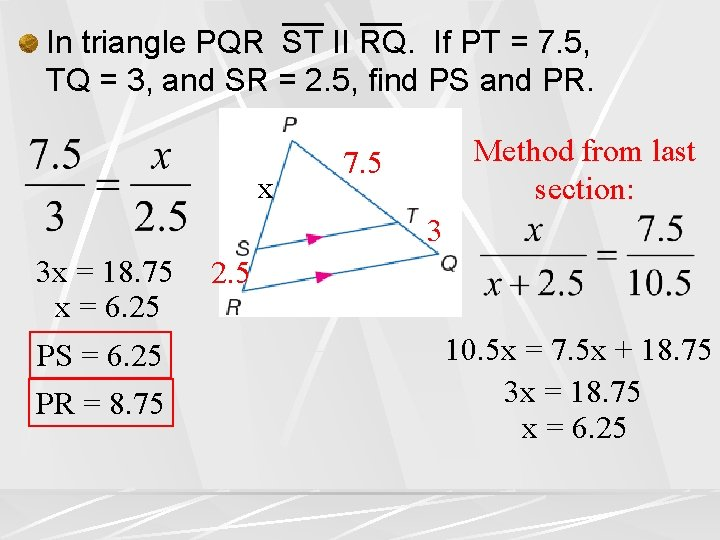In triangle PQR ST II RQ. If PT = 7. 5, TQ = 3, and SR = 2. 5, find PS and PR. x Method from last section: 7. 5 3 3 x = 18. 75 x = 6. 25 PS = 6. 25 PR = 8. 75 2. 5 10. 5 x = 7. 5 x + 18. 75 3 x = 18. 75 x = 6. 25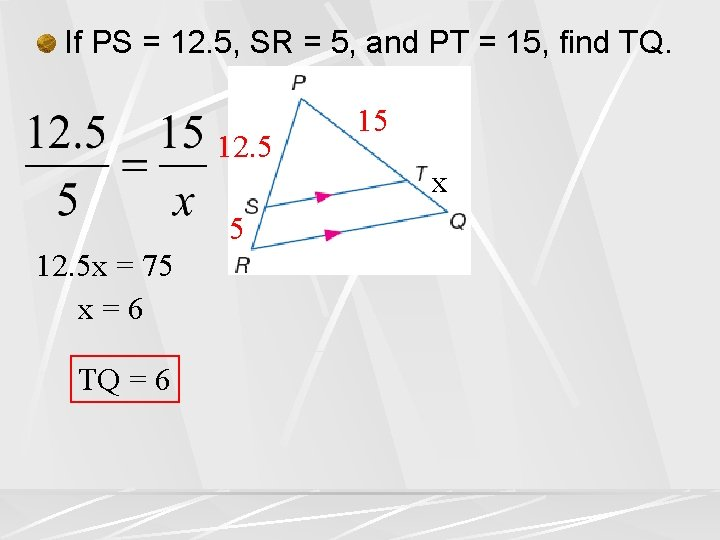If PS = 12. 5, SR = 5, and PT = 15, find TQ. 12. 5 5 12. 5 x = 75 x=6 TQ = 6 15 xTheorem Converse of Triangle Proportionality Theorem l If a line intersects two sides of a triangle and separates the sides into proportional corresponding segments, then the line is parallel to the third side of the triangle.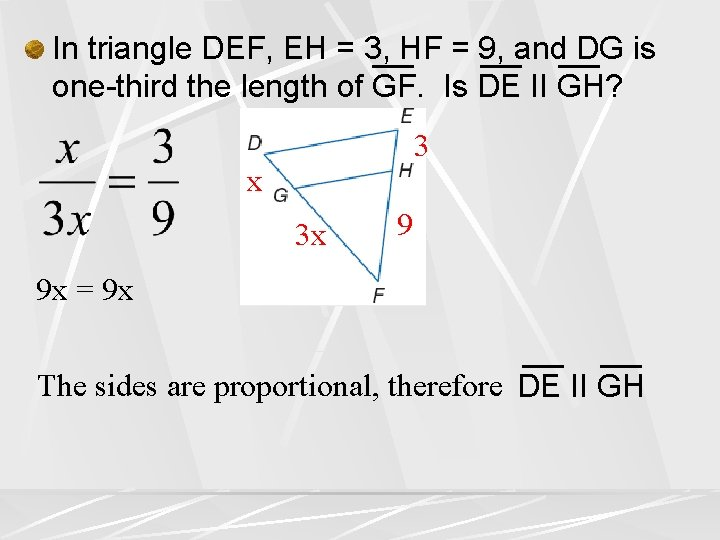In triangle DEF, EH = 3, HF = 9, and DG is one-third the length of GF. Is DE II GH? 3 x 3 x 9 9 x = 9 x The sides are proportional, therefore DE II GH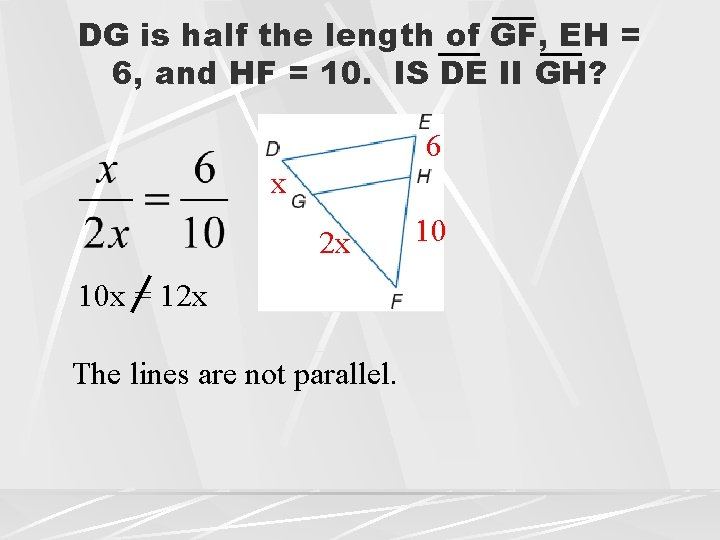DG is half the length of GF, EH = 6, and HF = 10. IS DE II GH? 6 x 2 x 10 x = 12 x The lines are not parallel. 10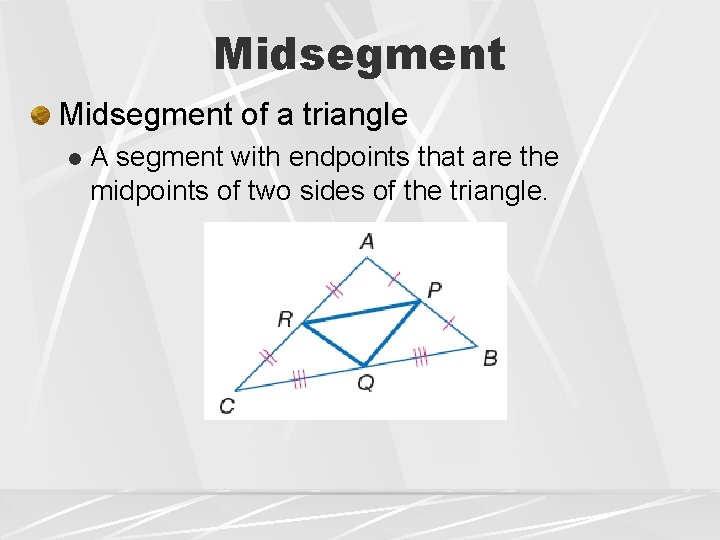Midsegment of a triangle l A segment with endpoints that are the midpoints of two sides of the triangle.Theorem Triangle Midsegment Theorem l A midsegment of a triangle is parallel to one side of the triangle, and its length is one half the length of that side.XY and XZ are midsegments of the triangle. Find XZ 6. 5 ST 7 6. 5 14 124 o 6. 5 7 6. 5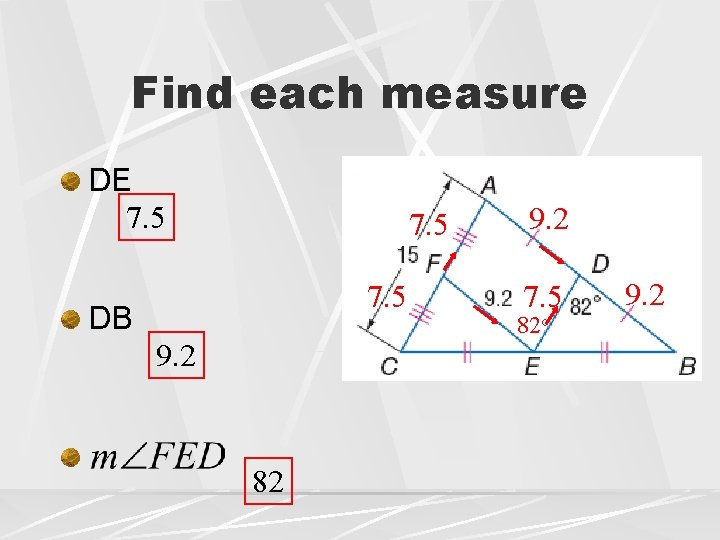Find each measure DE 7. 5 DB 9. 2 82 9. 2 7. 5 82 o 9. 2Proportional Parts of Parallel Lines If three or more parallel lines intersect two transversals, then they cut off the transversals proportionally.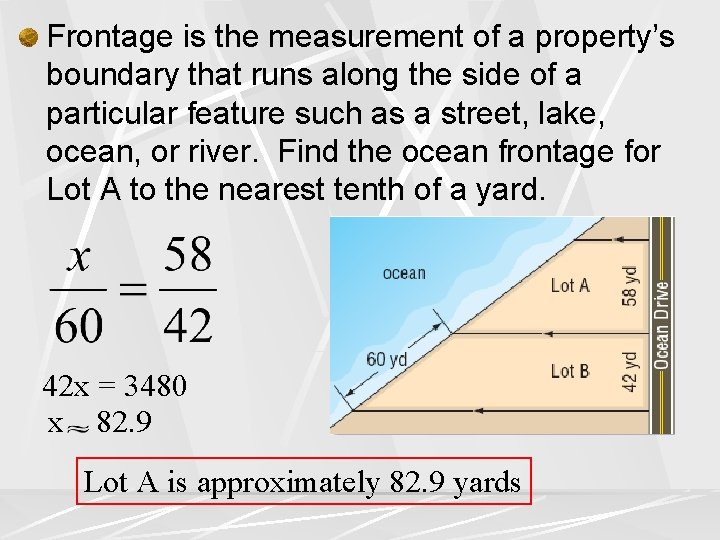Frontage is the measurement of a property’s boundary that runs along the side of a particular feature such as a street, lake, ocean, or river. Find the ocean frontage for Lot A to the nearest tenth of a yard. 42 x = 3480 x 82. 9 Lot A is approximately 82. 9 yards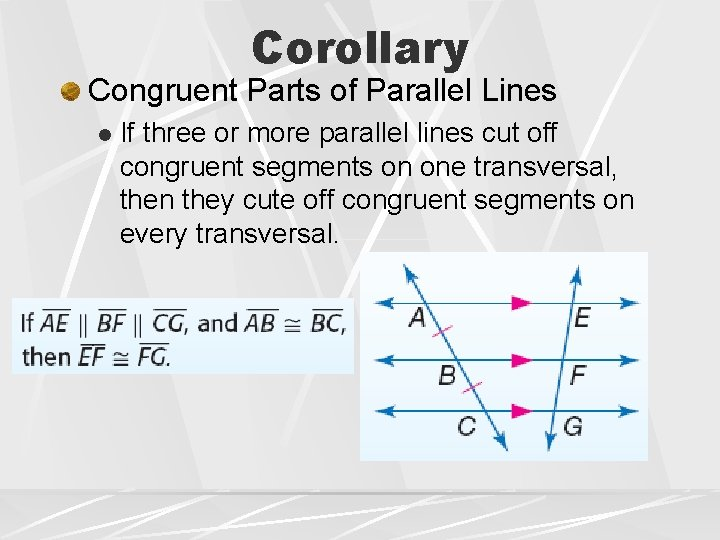Corollary Congruent Parts of Parallel Lines l If three or more parallel lines cut off congruent segments on one transversal, then they cute off congruent segments on every transversal.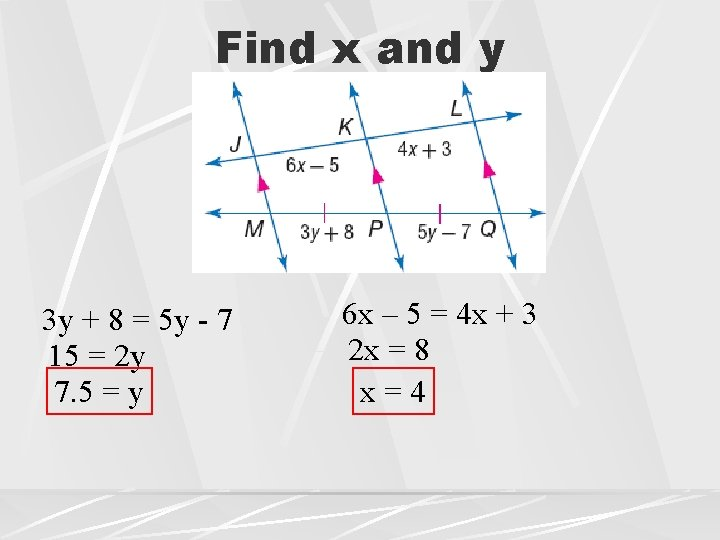Find x and y 3 y + 8 = 5 y - 7 15 = 2 y 7. 5 = y 6 x – 5 = 4 x + 3 2 x = 8 x=4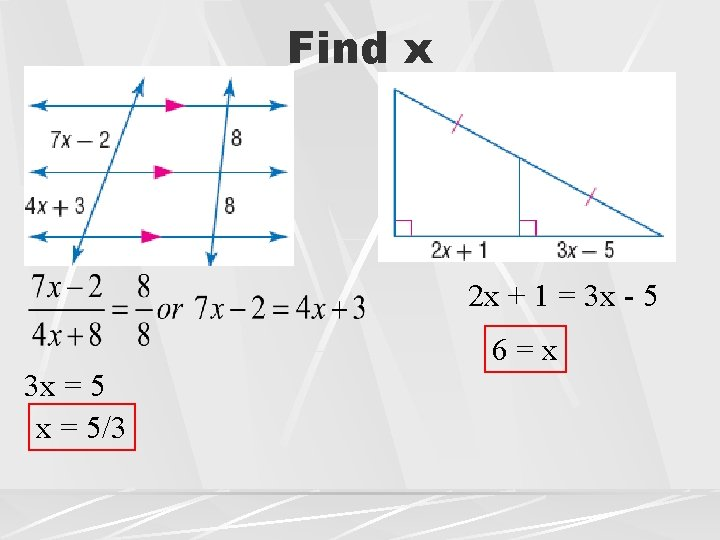Find x 2 x + 1 = 3 x - 5 6=x 3 x = 5/3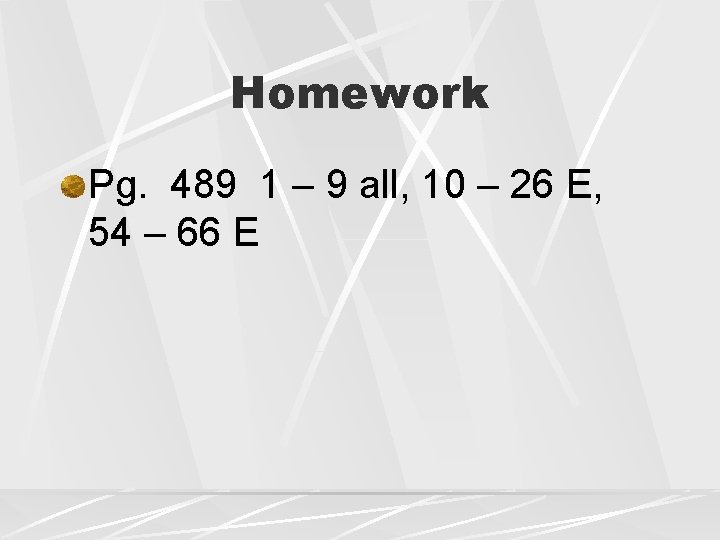Homework Pg. 489 1 – 9 all, 10 – 26 E, 54 – 66 E